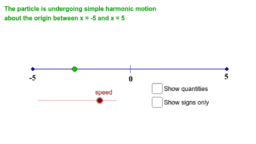•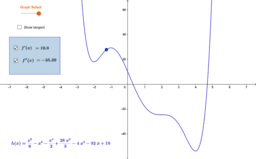The First and Second Derivatives

Activity

•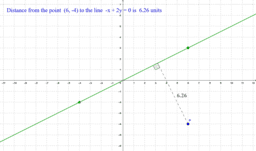Distance from a point to a line

Activity

•The meaning of indices

Activity

•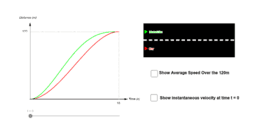The car and the motorbike

Activity

•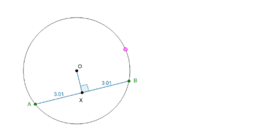Chord Properties 1

Activity

•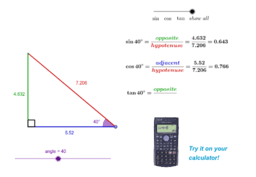sin, cos and tan in a right angled triangle

Activity

•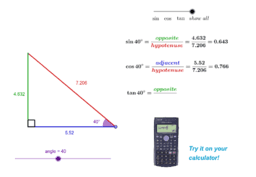Ratio of sides in a right-angled triangle

Activity

•Index laws - multiplication

Activity

•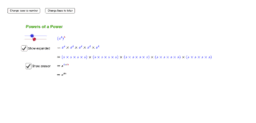Index laws - power of a power

Activity

•Acute and Obtuse angle relationships on the unit circle

Activity

•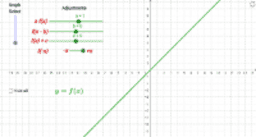Transformations of Functions

Activity

•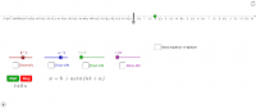Simple Harmonic Motion

Activity

•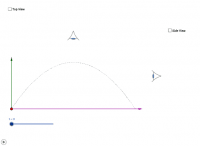Projectile Motion - Components of Motion

Activity

•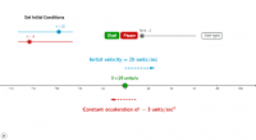Initial velocity and acceleration

Activity

•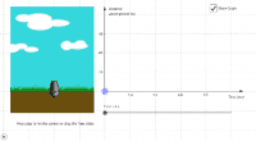The Cannon

Activity

•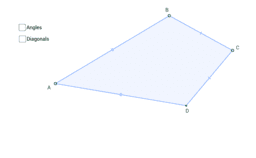Properties of a Kite

Activity

•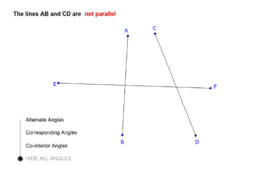Parallel line relationships

Activity

•Graphs of sin and cos

Activity

•Exact values on the unit circle (degrees)

Activity

•Arcs, Angles and Chords Theorem 2

Activity

•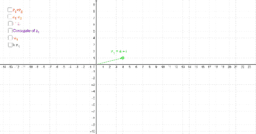Operations on complex numbers as vectors

Activity

•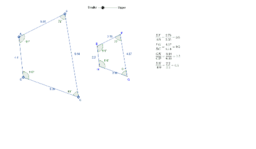Investigating similar figures

Activity

•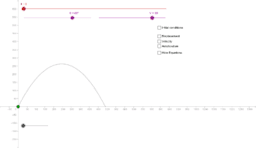Projectile Motion

Activity

•